# Bridge piers

One quarter of the bridge pier is sunk into the ground. Two thirds are in the water. Protruding above the water is 1.20 m long. Determine the height of bridge piers.

x =  14.4 m

### Step-by-step explanation:

x/4+2/3 x + 1.2 = x

x/4+2/3•x + 1.2 = x

x = 14.4

x = 72/5 = 14.4

Our simple equation calculator calculates it.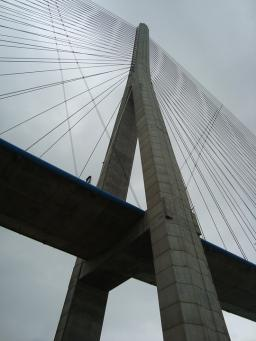Did you find an error or inaccuracy? Feel free to write us. Thank you!Tips to related online calculators
Need help to calculate sum, simplify or multiply fractions? Try our fraction calculator.
Do you have a linear equation or system of equations and looking for its solution? Or do you have a quadratic equation?
Do you want to convert length units?

## Related math problems and questions:

• Two thirdsFind two-thirds of the number equal to two-thirds of 99
• Iron pole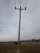The iron pole is in the ground 2/5 of its length, partly above the ground 1/3 is yellow, and the unpainted section is 6 m long. How long is the entire column?
• Quarter circularThe wire that is hooked around the perimeter of quarter-circular arc has length 3π+12. Determine the radius of circle arc.
• A bridgeA bridge over a river has the shape of the arc with bases of the bridge at the river's edge. At the center of the river, the bridge is 10 feet above the water. At 27 feet from the edge of the river, the bridge is 9 feet above the water. How wide is the ri
• RainfallA rectangular garden of 25m in length and width 20m in width fall 4mm of water. Express by a fraction in basic form what part of the 60-hectolitre tank we would fill with this water.
• Charles Bridge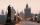Charles Bridge was 15 times older than Frantův bridge from 1900 in 1931. How old is the Charles Bridge?
• Water tank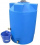The block-shaped tank is 2.5 m long, 100 cm wide and 12 dm high. In how many minutes will it be filled with water to two thirds if 40 liters of water flow into the tank per minute?
• New bridge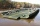Thanks to the new bridge, the road between A and B has been cut to one third and is now 10km long. How much did the road between A and B measure before?
• Water levelThe glass container has a cuboid shape with dimensions of the bottom 24 cm and 12 cm. The height of the water is 26 cm. Calculate the body's volume, which is sunk into the water if the water level rise by 3 cm.
• Long bridge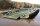Roman walked on the bridge. When he heard the whistle, he turned and saw running Kamil at the beginning of the bridge. If he went to him, they would meet in the middle of the bridge. Roman, however, rushed and so did not want to waste time returning 150m.
• Two trainsThrough the bridge, long l = 240m, the train passes through the constant speed at time t1 = 21s. A train running along the traffic lights at the edge of the bridge passes the same speed at t2 = 9s. a) What speed v did the train go? b) How long did it take
• Water tank300hl of water was filled into the tank 12 m long and 6 m wide. How high does it reach?
• Stones in aquariumIn an aquarium with a length 2 m; width 1.5 m and a depth of 2.5 m is a water level up to three-quarters of the depth. Can we place stones with a volume of 2 m3 into the aquarium without water being poured out?
• Bridge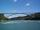The bridge arc has a span 34 m and height 3 m. Calculate the radius of the circle arc of this bridge.
• Warehouses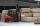To four warehouses is going cement in 25 kg bags. To first one third, to second quarter of the total. The third store got two thirds of the rest, and the last 310 tons came to fourth. How many cement is in all warehouses and how much got every one?
• Unknown number 6Determine x if 1/6 of x is equal to 2/5 of the number 24.
• Bamboo 2A piece of bamboo is to 2 1/4 meter long. Jessie cuts 1 1/3 meter to be used for making some kites and another piece 3/4 meter long to be given to his friend. How long is the me remaining piece of bamboo?